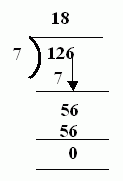Email us to get an instant 20% discount on highly effective K-12 Math & English kwizNET Programs!

#### Online Quiz (WorksheetABCD)

Questions Per Quiz = 2 4 6 8 10

### Grade 4 - Mathematics6.1 Estimating Quotients

 Method: Read the questions carefully. Divide and write the quotient and remainder if any using estimated method. Example: 126 ÷ 7 This can be estimated to 130 / 10 On dividing this we get 13, check this with the given problem. 13 x 7 = 91, choose a higher quotient. 14 x 7 = 98 15 x 7 = 105 16 x 7 = 112 18 x 7 = 126Answer: 18R0 Directions: Divide the following. Also write at least 10 examples of your own.
 Q 1: 36 ÷ 4Answer: Q 2: 12 ÷ 3Answer: Q 3: 24 ÷ 3Answer: Q 4: 42 ÷ 7Answer: Q 5: 66 ÷ 11Answer: Q 6: 88 ÷ 8Answer: Q 7: 96 ÷ 8Answer: Q 8: 84 ÷ 7Answer: Question 9: This question is available to subscribers only! Question 10: This question is available to subscribers only!